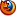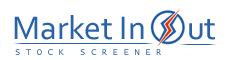Please enable JavaScript to view this page content properly Log In | Sign Up
 Pre-Tax Profit Margin Leverage Ratio Current Ratio Price / Book Ratio Price / Tangible Book Ratio Price / Cash Flow Ratio Price / Free Cash Flow Ratio Debt / Equity Ratio Price / Sales Ratio Income per Employee
 Leverage Ratio Action Leverage Ratio (Assets/Equity): More than 50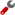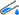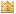Leverage Ratio (Assets/Equity): 20 to 50Leverage Ratio (Assets/Equity): 8 to 20Leverage Ratio (Assets/Equity): 5 to 8Leverage Ratio (Assets/Equity): 3 to 5Leverage Ratio (Assets/Equity): 2 to 3Leverage Ratio (Assets/Equity): 1 to 2Leverage Ratio (Assets/Equity): 0 to 1Best viewed in